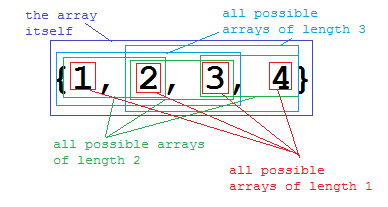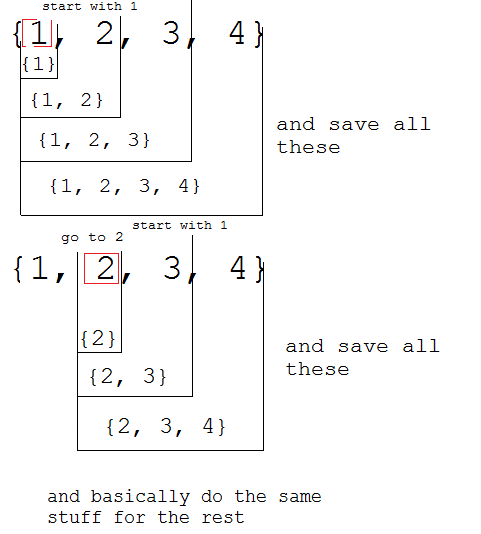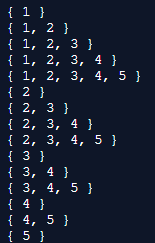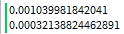# Coding Challenge #3: Continuous Subarrays

## Coding Challenge #3

Welcome back, to another coding challenge! I hope you have fun with today’s. I think it’s much simpler and shorter, and just requires some amount of creativity. Credit belongs to @Fifkee for sharing this challenge with me!

As usual, same requirements:

• Has to be written in Lua 5.1 (the version roblox uses)
• The program has to be compatible with Roblox Studio, meaning you can’t use features vanilla Lua has but rbxlua disabled
• You can’t use httpservice to cheat the challenge

Let’s say we have an array `A`.

We define `S` to be a subarray of `A` if contains some elements that also exist in `A`.

We define `S` to be a continuous subarray of `A`, if it is a subarray of `A`, and staisfies the condition: `S` , `S` , `...` , `S[n] = A[i]` , `A[i + 1]` , `...` , `A[i + n - 1]` for some index `i`. An array has multiple continuous arrays.

For this challenge, you need to create a function `subarrays`, which takes an array as input, and return a table containing all of the array’s continuous subarrays.

Wait… What? Well the first part is simple, but what about the continuous subarray? I think giving an example is the best way to explain it.

Let `A` be `{1, 2, 3}`, the continuous subarrays of `A` would be `{}`, `{1}`, `{2}` , `{3}` , `{1, 2}` , `{2, 3}` and `{1, 2, 3}`.

Let `A` be `{1, 2, 3, 4}`, the continuous subarrays of `A` would be `{}`, `{1}`, `{2}` , `{3}` , `{4}`, `{1, 2}` , `{2, 3}`, `{3, 4}`, `{1, 2, 3}`, `{2, 3, 4}` and `{1, 2, 3}`.Basically, you need to make all possible subarrays using initial array A’s elements that have a length of 1, and all subarrays that have a length of 2, where the elements of each subarray are next to each other (e.g `{1, 3}` isn’t correct, because 1 and 3 aren’t next to each other) ect.

Anywho, this is it again! I hope you have fun with this one! You can find a list of all the challenges here or a github version here.The answer is here, don't spoil yourself!

Basically, the way this will work is, we will loop through the array, and for each element, using the fact that `unpack(table, from, to)` has two optional argument; from where to start and stop unpacking, we loop from that element to the next element, inserting those two into a subarray, then loop from the same element to the next next element ect. until the there are no elements, and we move to the second element, and do the same stuff.``````local function subarrays(array) --array is the inputted array
local subarrays = {{}} --this is the returned table, {} is considered a subarray as well
for x, _ in pairs(array) do --loop through all elements
for i = x, #array do --loop from the element's index, to the end of the list, i would be the current next element
table.insert(subarrays, {unpack(array, x, i)}) --basically insert an array in the returned table of elements starting from the element and the current next element
end
end
return subarrays --return
end

local A = {1,2,3,4,5} --an example

for i, v in pairs(subarrays(A)) do
print(string.format("{ %s }", table.concat(v, ", "))) --just a way to print the subarrays
end
``````

Result:Note that order isn’t needed.

13 Likes

Still forgetting subarray `{}`, Starma.imeanitismycodesotechnicallyiforgotitbutstill

2 Likes

I got confused and thought that you only wanted consecutive elements that were consecutive. (For example, {1, 2, 4, 3, 5} would have {1, 2} as the longest since 3 doesn’t come after 4 and 5 doesn’t come right after 3.) Since your examples were all 1, 2, 3, 4 and stuff like that, it was hard to tell that’s not what you were asking for. Here’s my code anyways:

code
``````local function findSubsection(array, start, stop)
-- note: doesn't deep copy (as u can see lol)
local final, j = {}, 1

for i = start, stop do
final[j] = array[i]
j = j + 1
end

return final
end

return function(array)
local last = {};
local subarrays = {{},} -- haha unlike Fifkee i did not forget the empty table

for _, value in ipairs(array) do
subarrays[#subarrays + 1] = {value} -- insert the 1 element subarray
print(value, repr(last))

if last[#last] == value - 1 then -- if it's sequential with the last
local subsection
-- probably an awful method (should really be local to scope of 2nd `for`
-- but i want the last subsection to become the new `last`

for i = 1, #last do
subsection = findSubsection(last, i, #last)
subsection[#subsection + 1] = value

subarrays[#subarrays + 1] = subsection
end

last = subsection
else
last = {value}
end
end

return subarrays
end
``````

Ozzypig’s Repr made this super easy to test.

Here’s my reworked code now that I actually understand what you’re asking for. I found this out after looking at (and testing) your solution, so it’ll probably be pretty similar. (I hadn’t wrote mine yet at the time of writing this.)

reworked code
``````local function findSubsection(array, start, stop)
-- note: doesn't deep copy (as u can see lol)
local final, j = {}, 1

for i = start, stop do
final[j] = array[i]
j = j + 1
end

return final
end

return function(array)
local last = {};
local subarrays = {{},} -- haha unlike Fifkee i did not forget the empty table

for start = 1, #array do
for stop = start, #array do
subarrays[#subarrays + 1] = findSubsection(array, start, stop)
end
end

table.sort(subarrays, function(subtable1, subtable2) -- bonus! since i already saw solution
local length1, length2 = #subtable1, #subtable2

if length1 ~= length2 then
return length1 < length2
else
return subtable1 < subtable2
end
end)

return subarrays
end
``````
6 Likes

Very great! As usual!

From the looks of it, it seems to work.

Also I assume it’s a module script? Because you’re returning the function.

I really like how yor attempt is different then the one in the challenge (which is @Fifkee’s btw).

3 Likes

Yeah, I forgot to mention that. They’re both ModuleScripts.

3 Likes

Submitted by @Abandion!

``````arr = {1,2,3,4,5,6,7,8,9}

function gensubarrays(array)
ret = {}
length = #array

for i = 2, length do
for h = 1, (length - (i-1)) do
to = {}
for j = 1, i do
table.insert(to, array[h + (j-1)])
end
table.insert(ret, to)
end
end

return ret
end

for i, v in pairs(gensubarrays(arr)) do
a = ""
for i, v in pairs(v) do a = a..v end
print(a)
end
``````
1 Like

i made this challenge heres my sol (solution btw)

``````local function generateSubarrays(arr)
local subarrays = {{}}

for size = 1, #arr do
for start = 1, #arr - size + 1 do
local fin = start + size - 1

table.insert(
subarrays,
{ unpack(arr, start, fin) }
)
end
end

return subarrays
end
``````
2 Likes
``````local function subarrays(array)
local length = #array
local s = table.create(length*(length+1)/2 - 1, {})
local i = 1
for n=1, length - 1 do
for j=1, length - n + 1 do
s[i] = table.move(array, j, j + n - 1, 1, table.create(n, 0))
i = i + 1
end
end
return s
end
``````
``````{ 1 }
{ 2 }
{ 3 }
{ 4 }
{ 5 }
{ 1, 2 }
{ 2, 3 }
{ 3, 4 }
{ 4, 5 }
{ 1, 2, 3 }
{ 2, 3, 4 }
{ 3, 4, 5 }
{ 1, 2, 3, 4 }
{ 2, 3, 4, 5 }
``````
2 Likes

A solution using recursion:

``````local function q(a, o, i, j)
o = o or {{}}
i = i or 1
j = j or 1
if j > #a then
return o
elseif i > j then
return q(a, o, 1, j + 1)
end
o[#o + 1] = {unpack(a, i, j)}
return q(a, o, i + 1, j)
end
``````
``````{  }
{ 1 }
{ 1, 2 }
{ 2 }
{ 1, 2, 3 }
{ 2, 3 }
{ 3 }
{ 1, 2, 3, 4 }
{ 2, 3, 4 }
{ 3, 4 }
{ 4 }
{ 1, 2, 3, 4, 5 }
{ 2, 3, 4, 5 }
{ 3, 4, 5 }
{ 4, 5 }
{ 5 }
``````
4 Likes

Good idea using `table.create` for allocating since we know the size of the array. It seems to be faster than mine!

``````local start1 = tick()
for i = 1, 100 do
local random = table.create(math.random(1, 10), 5) --array of random size
local a = subarrays(random)--my algorithm
end
print(tick()-start1)

local start2 = tick()
for i = 1, 100 do
local random = table.create(math.random(1, 10), 5) --array of random size
local a = subarrays2(random) --your algorithm
end
print(tick()-start2)
``````1 LikeA subarray is simply a part of an array. If you have an array of size `#t`, a subarray is any array `{unpack(t, i, j)}` such as `0 < i <= j <= #t`, including the empty array `{}`.
Or simply, if you have the array `{1, 2, 3, 4}`, `{1, 2}` is a subarray, `{2, 3}` is a subarray, `{2, 3, 4}` is a subarray, `{1}` is a subarray etc. even `{1, 2, 3, 4}` is a subarray of itself. So really you pick a starting index, and choose an end index within the bounds of the array, that’s a subarray. The only special case is `{}` which is a subarray of all arrays. The challenge is to return all the subarrays of a given array.